Courses

# NCERT Exemplar - Some Application of Trigonometry Class 10 Notes | EduRev

## Class 10 : NCERT Exemplar - Some Application of Trigonometry Class 10 Notes | EduRev

The document NCERT Exemplar - Some Application of Trigonometry Class 10 Notes | EduRev is a part of the Class 10 Course Mathematics (Maths) Class 10.
All you need of Class 10 at this link: Class 10

Q.1. If a pole 6 m high casts a shadow 2√3 m long on the ground, find the Sun’s elevation.
Ans.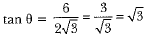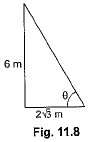⇒ θ = 60°

Q.2. If the angle of elevation of a tower from a distance of 100 m from its foot is 60°, then what will be the height of the tower?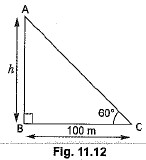Ans.
Let h be the height of the tower.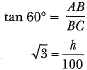h = 100√3 m

Q.3. A spherical balloon of radius r subtends an angle θ at the eye of an observer. If the angle of elevation of its centre is φ, find the height of the centre of the balloon.
Ans.
In Fig. 11.55, O is the centre of balloon, whose radius OP = r and ∠PAQ = θ. Also, ∠OAB = φ. Let the height of the centre of the balloon be h. Thus OB = h.
In ΔOAP, we have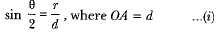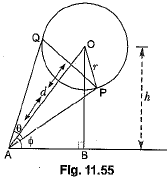Also in ΔOAB,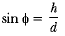...(ii)
From (i) and (ii), we get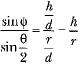or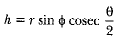Q.4. If the angle of elevation of a cloud from a point h metres above a lake is α and the angle of depression of its reflection in the lake is β, prove that the height of the cloud is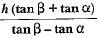Ans.
Let AB be the surface of the lake and let P be a point of observation (Fig. 11.58) such that AP = h metres. Let C be the position of the cloud and C' be its reflection in the lake. Then, CB = C'B. Let PM be perpendicular from P on CB. Then, ∠CPM = α and ∠MPC‘ = β. Let CM = x.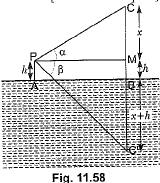Then, CB = CM + MB = CM + PA = x + h
In ΔCPM, we have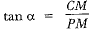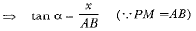⇒ AB = x cot α
In ΔPMC’, we have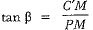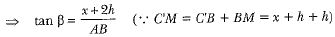⇒ AB = (x + 2h) cotβ ...(ii)
From (i) and (ii), we have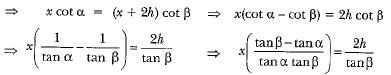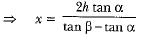Hence, the height CB of the cloud is given by
CB = x + h ⇒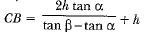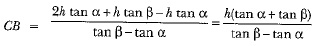Q.5. The lower window of a house is at a height of 2 m above the ground and its upper window is 4 m vertically above the lower window. At certain instant the angles of elevation of a balloon from these windows are observed to be 60° and 30° respectively. Find the height of the balloon above the ground.
Ans.
Let height of balloon = h m
In rt. ΔGDC,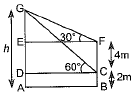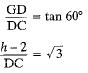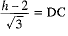...(i)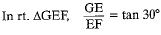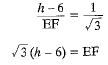According to question
DC = EF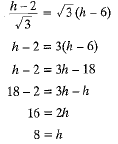Height of the balloon above the ground is 8 m.

Offer running on EduRev: Apply code STAYHOME200 to get INR 200 off on our premium plan EduRev Infinity!

## Mathematics (Maths) Class 10

51 videos|346 docs|103 tests

,

,

,

,

,

,

,

,

,

,

,

,

,

,

,

,

,

,

,

,

,

;